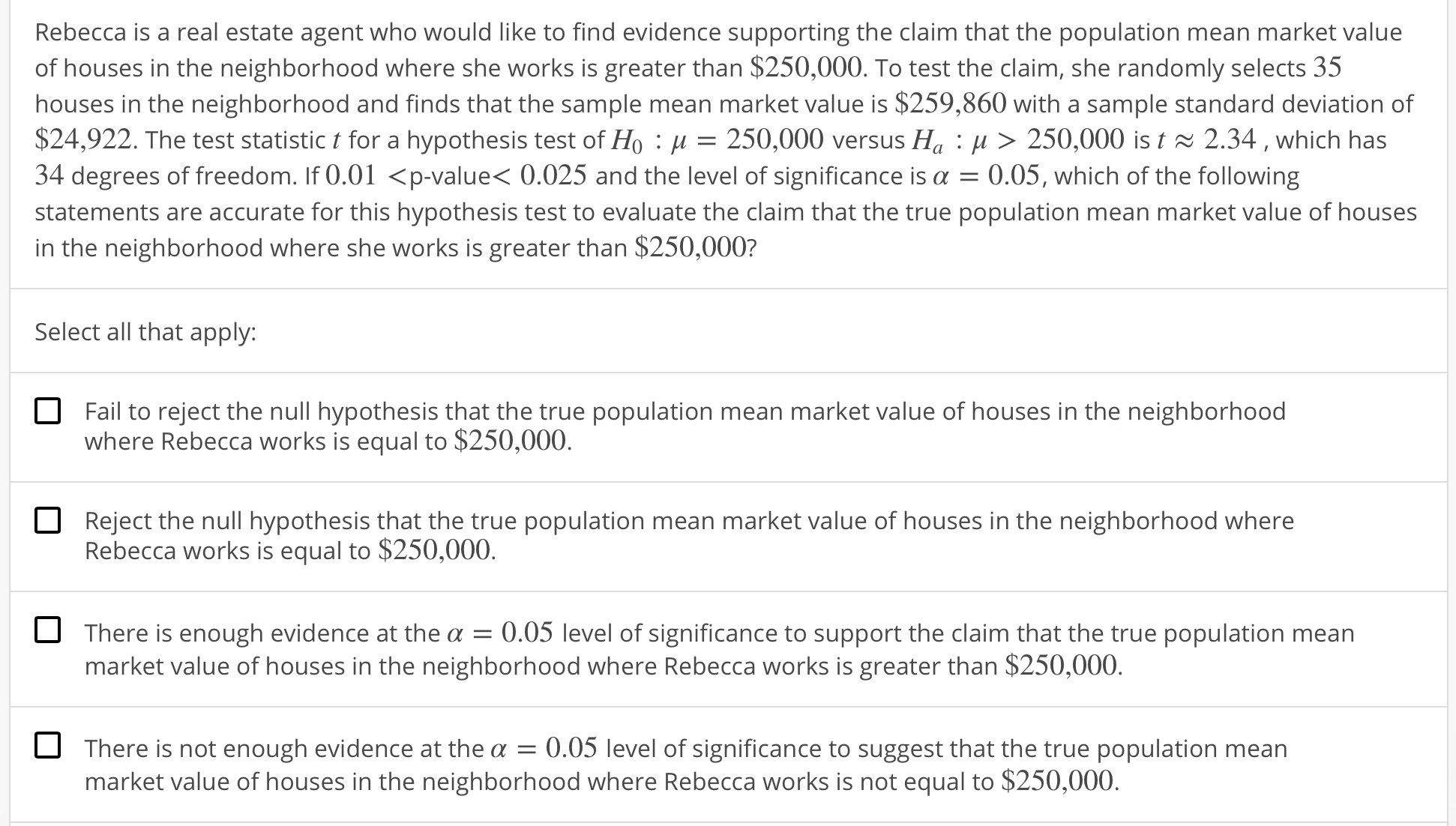# Rebecca is a real estate agent who would like to find evidence supporting the claim that the population mean market valueof houses in the neighborhood where she works is greater than \$250,000. To test the claim, she randomly selects 35houses in the neighborhood and finds that the sample mean market value is \$259,860 with a sample standard deviation of\$24,922. The test statistic t for a hypothesis test of Ho : μ = 250,000 versus Ha : μ 〉 250,000 is t 2.34 , which has34 degrees of freedom. If 0.01

Question
85 viewshelp_outlineImage TranscriptioncloseRebecca is a real estate agent who would like to find evidence supporting the claim that the population mean market value of houses in the neighborhood where she works is greater than \$250,000. To test the claim, she randomly selects 35 houses in the neighborhood and finds that the sample mean market value is \$259,860 with a sample standard deviation of \$24,922. The test statistic t for a hypothesis test of Ho : μ = 250,000 versus Ha : μ 〉 250,000 is t 2.34 , which has 34 degrees of freedom. If 0.01
check_circle

Step 1

Test hypothesis:

Denote the population mean market value of houses in the neighborhood of Rebecca as μ.

The investigator is interested to test whether the population mean market value of houses in the neighborhood of Rebecca is greater than \$250,000 or not.

The hypotheses are given below:

Null hypothesis:

H0 : μ = \$250,000

That is, the population mean market value of houses in the neighborhood of Rebecca is equal to \$250,000.

Alternative hypothesis:

Ha : μ > \$250,000 (Right tail test).

That is, the population mean market value of houses in the neighborhood of Rebecca is greater than \$250,000.

Step 2

Given information.

Here, the population standard deviation is not known.

Under null hypothesis the population mean market value of houses in the neighborhood of Rebecca is μ = \$250,000.

The sample mean market value of houses in the neighborhood of Rebecca is x-bar = \$259,860, the sample size is n = 35 and the sample standard deviation in the market value of houses in the neighborhood of Rebecca is s = \$24,992.

The test statistic value is t = 2.34 and the P–value lies between 0.01 and 0.025 and the level of significance is α = 0.05.

Step 3

Decision rule:

Decision rule based on P-value approach:

If P-value ≤ α, then reject the null hypothesis H0.

If P-value >...

### Want to see the full answer?

See Solution

#### Want to see this answer and more?

Solutions are written by subject experts who are available 24/7. Questions are typically answered within 1 hour.*

See Solution
*Response times may vary by subject and question.
Tagged in

### Hypothesis Testing